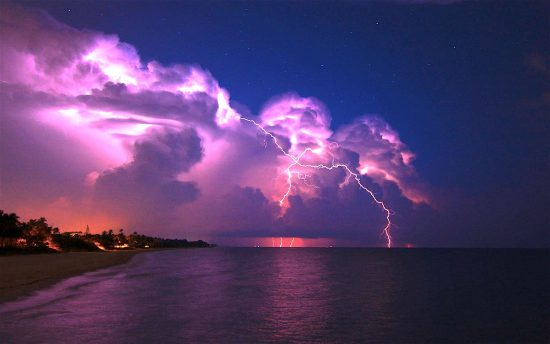# Lightning’s Power Part OnePublic access image of a thunderstorm.

Apr 23, 2018

What is lightning’s source?

Electric discharges in plasma create electromagnetic sheaths along their axes. If the charge flow is high enough, sheaths will glow, sometimes creating a number of other sheaths. The sheaths are called “double layers”, and powerful electric fields appear between regions, which can accelerate charged particles.

Whenever charged particles are in motion, electricity is generated, which is defined as electric current, or a flow of electric charge through a medium. Ordinarily, that unit of charge is referred to simply as a “coulomb”, although, in reality, there are two forms of charge: electrostatic units of charge (statcoulomb) and electromagnetic units (abcoulomb). One coulomb of electric charge equals about 3,000,000,000 electrostatic units, or one-tenth of an electromagnetic unit. In Electric Universe models the concern is primarily abcoulombs, so in any calculations involving the activity of electricity in space, it can be assumed that electromagnetic charge is meant when “coulomb” is used.

The flow of electric charge per second is an “ampere”, symbolized by the letter A. The ampere is a measure of the amount of electric charge passing a particular point in any given time, but it is usually expressed in seconds.

When electric charges are separated, an electric potential exists between the two regions of charge (in a storm cloud, for example). For one ampere of charge (usually referred to as Q) to flow in a conductor with one ohm of resistance requires “electromagnetic force” of one volt. The work necessary to drive an electric charge of one coulomb across a potential difference of one volt in any given time is a joule. One volt is equivalent to one joule per coulomb.

In order to calculate electromagnetic forces, Newtons (N), Joules (J), and Coulombs (C) are used. Electric field measurements, for instance, are obtained by dividing force and potential energy by charge: Newtons divided by coulombs (N/C) and joules divided by coulombs (J/C). Since a joule per coulomb is a volt (V), electric potential is normally called voltage. So, electric fields (E) are quoted in volts per meter (M), since V/M = N/C.

Earth is an electrically charged body and holds an average electric field at its surface of 50 – 200 volts per meter. That electricity arrives in the form of ionic particles emitted by the Sun, called the “solar wind” that speeds along massive electric circuits called “Birkeland currents” that have been discussed many times in these pages.

Electric Universe theory sees clouds acting like the plates in a capacitor, storing electric charge. Observations show that there is a “wind” of charged particles blowing toward any developing thunderstorm, which could be interpreted as an electric current at the cloud base. Surrounding air molecules get pulled along with the current flow, forming powerful updrafts that can rise into the stratosphere. The capacitor effect is probably what contributes to lightning discharges.

Capacitors are two conductors separated by an insulating medium, or “dielectric insulator”. An electric charge on one conductor attracts an opposite charge to the other conductor, resulting in an electric field that stores electrical energy. Thunderstorms are most likely behaving like capacitors: clouds are one plate, the ground another, and the atmosphere is the dielectric insulator. Cloud bases usually acquire negative charge and the cloud tops are positive, although that can be the opposite case.

The Time History of Events and Macroscale Interactions during Substorms (THEMIS) satellites found electrified plasma vortices rotating faster than 1,600,000 kilometers per hour, about 64,000 kilometers from Earth. That phenomenon is familiar to Electric Universe advocate. They are called, Birkeland currents. The THEMIS satellites, together with Earth-based stations, verified that those charged plasma formations are connected to the ionosphere. That means the Sun is directly coupled to thunderstorms.

Since thunderstorms are connected to an electric circuit that couples to the ionosphere, electric charge from the solar wind increases electrical energy in the clouds, which also increases the stored charge in the ground. That accumulated charge eventually overcomes the atmosphere’s ability to keep electric potentials separated, so electrons reach out from the clouds to the ground in the form of “leader strokes”.

Leader strokes descend from the clouds at hundreds of meters per second, forming ionized channels in the atmosphere that are far more conductive than their surroundings. The ability for electricity to move within those filaments, sometimes several meters wide, can require many orders of magnitude less work than that needed to initiate the dielectric breakdown.

At a height of one kilometer, the electric field beneath a strong thunderstorm can reach 3 million volts per meter (3×10^6vm), until a dielectric breakdown occurs with an accompanying electron cascade. Electric potential beneath a thunderstorm can vary due to many factors, but is going to be between 3×10^7 volts and 1.5×10^8 volts.

With that information, assuming an ideal thunderstorm at one kilometer altitude (d), whose cloud tops are ten kilometers high, and covers a ground track of five kilometers, the potential difference between the cloud and the ground (∆v) is the electric field (E) times the distance:

∆v = Ed

If the dielectric breakdown occurs at 3×10^6 vm (for E) , and the distance between the cloud and the ground of 1 kilometer equals 10^3 meters, then solving,

∆v = Ed = 10^6 x 10^3 = 10^9 vm or, one billion volts.

However, it is not possible to accurately extrapolate these ideals into the real world, because conditions in nature are not uniform or consistent. Electric discharges can move in electric fields, as mentioned above, with orders of magnitude less effort than required to initiate the discharge. That means that any discharge beyond the meter scale is nearly impossible to estimate, although 10^9 volts x 10^5 amperes, or 10^14 watts is not uncommon.

Next, how those calculations might scale-up.

Stephen Smith